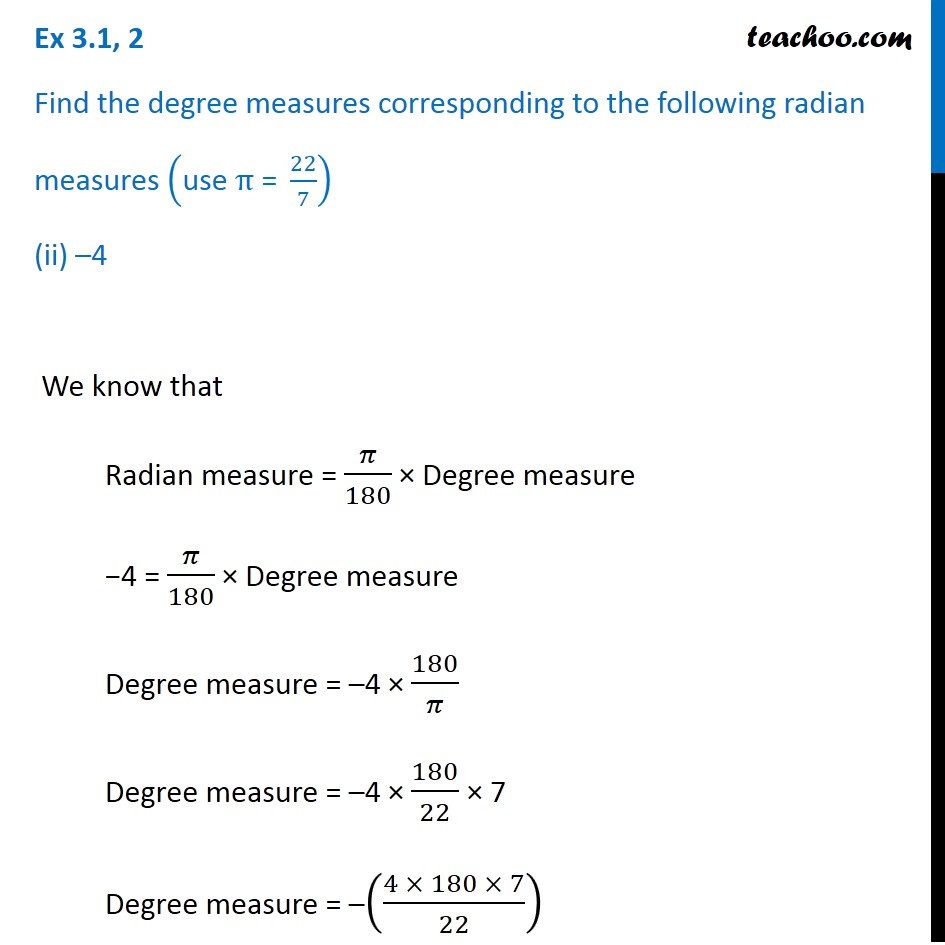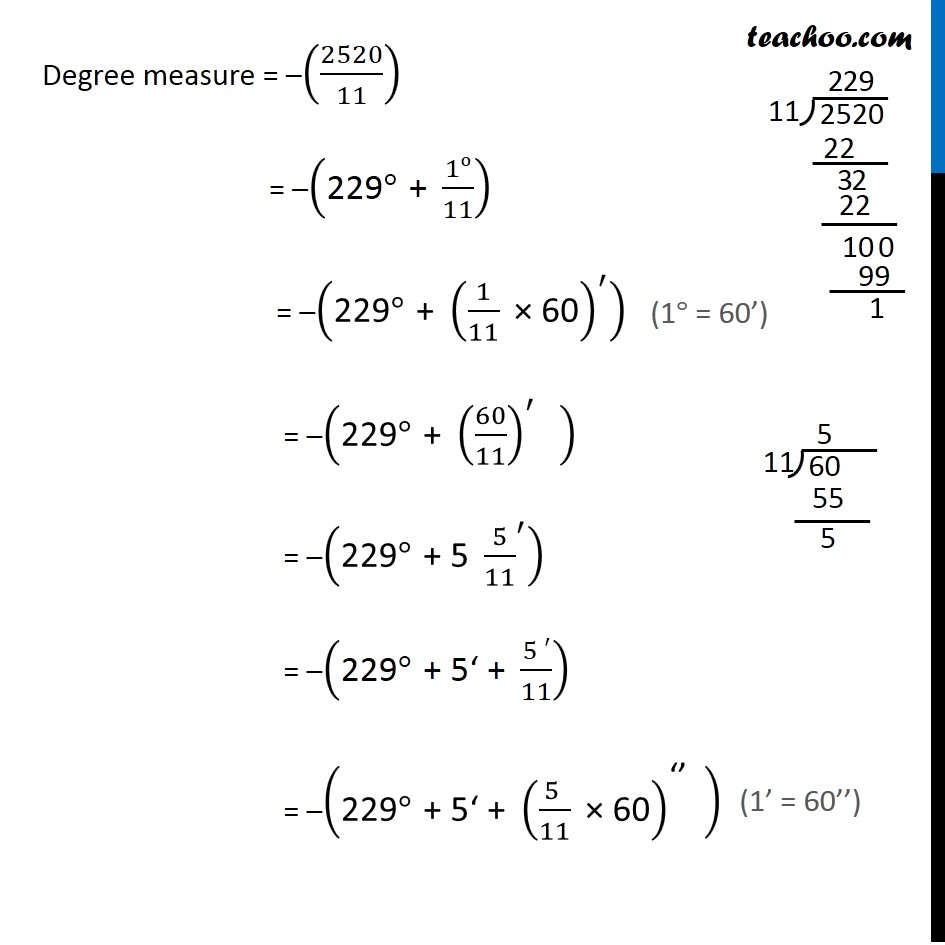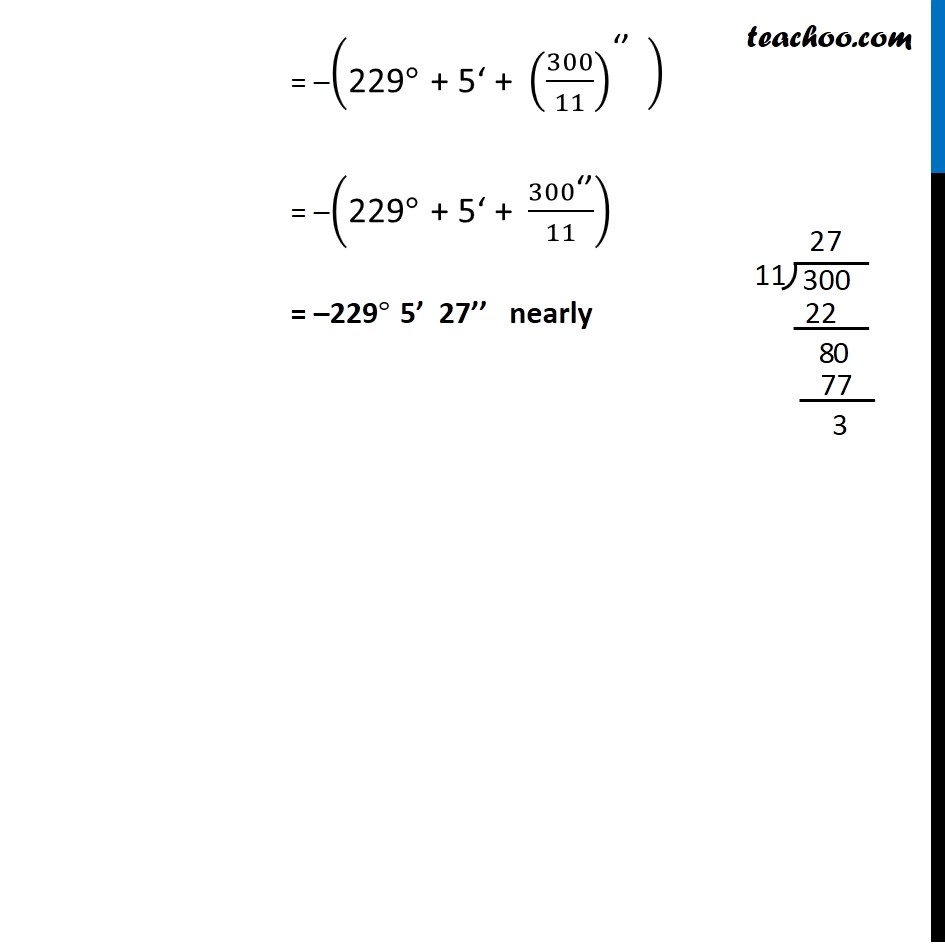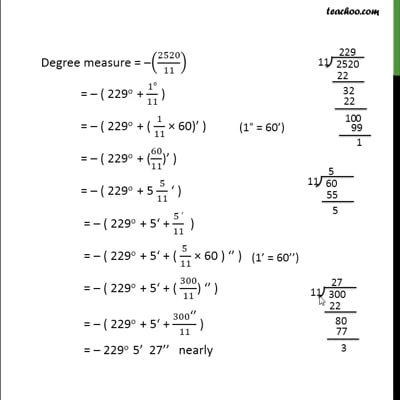Ex 3.1

Chapter 3 Class 11 Trigonometric Functions (Term 2)
Serial order wiseThis video is only available for Teachoo black users

### Transcript

Ex 3.1, 2 Find the degree measures corresponding to the following radian measures ("use " π" = " 22/7) (ii) –4 We know that Radian measure = 𝜋/180 × Degree measure −4 = 𝜋/180 × Degree measure Degree measure = –4 × 180/𝜋 Degree measure = –4 × 180/22 × 7 Degree measure = –((4 × 180 × 7)/22) Degree measure = –(2520/11) = –("229" °" + " 1o/11) = –("229" °" + " (1/11 " × 60" )^′ ) = –("229" °" + " (60/11)^′ " " ) = –("229" °" + 5 " 〖5/11〗^′ ) = –("229" °" + 5‘ + " (5 ′)/11) = –("229" °" + 5‘ + " ((5 )/11 " × 60" )^"‘’" " " ) = –("229" °" + 5‘ + " (300/11)^"‘’" " " ) = –("229" °" + 5‘ + " 300"‘’" /11) = –229° 5’ 27’’ nearly